Page No. 1033

Math / Math Worksheets / Fraction Worksheets

Download free printable fraction worksheets in PDF. Our math fractions worksheets are generated automatically, so you get an unlimited number of fraction sheets in PDF.

Print out free fractions worksheets and use them in the classroom or for home practice.

Find our online fraction games here →

## Printable Fraction Worksheets in PDF

Our fraction math worksheets contain also a link to the online fraction game, so kids can practice fractions without printing out the fraction worksheet. New free fractions worksheets will be added soon.

### Identifying fractions

What is the fraction of the shaded part?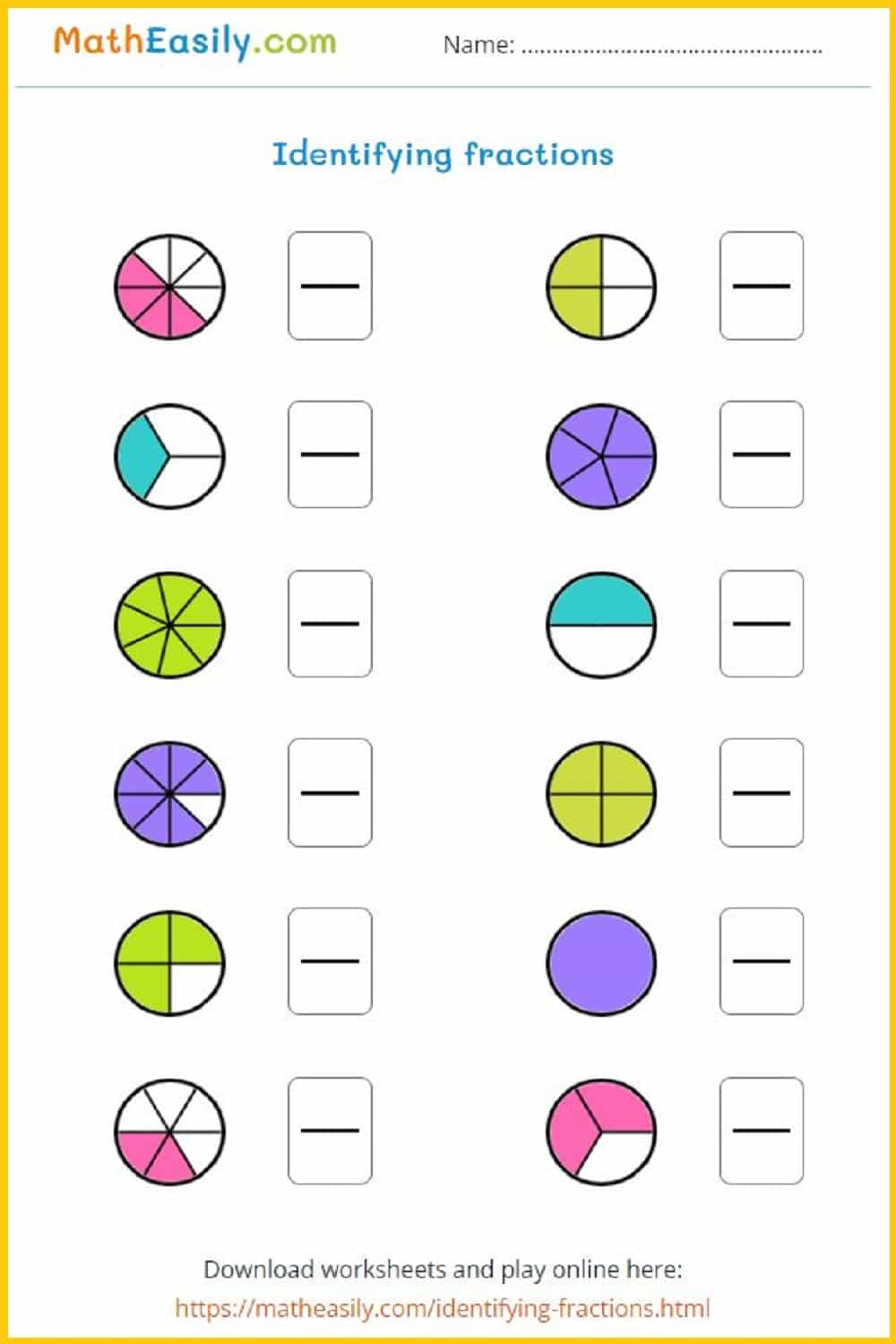Generate a new PDF

### Fraction identification

Color each circle to show the equivalent fraction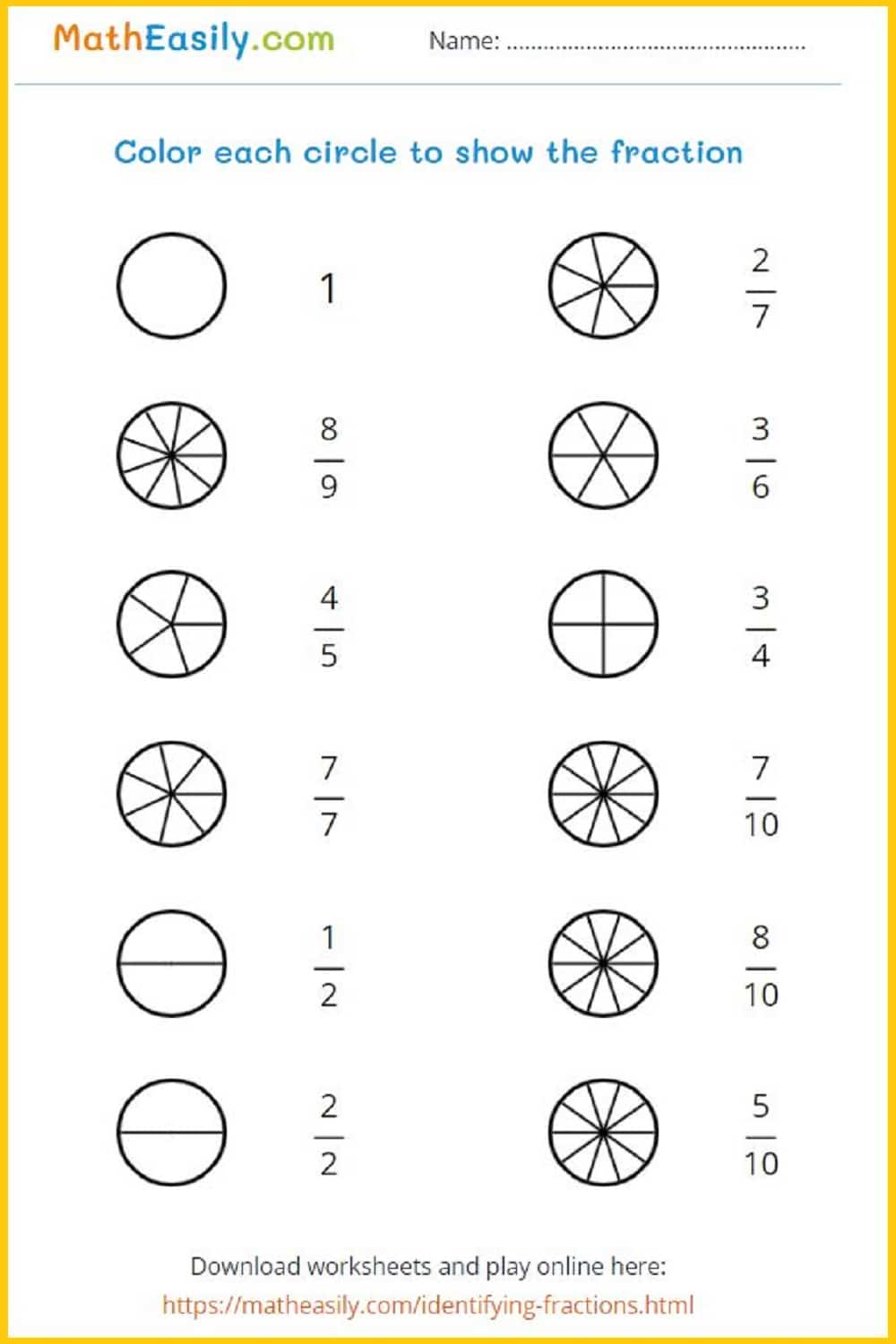Generate a new PDF

### Identify fractions

Circle the correct fraction from the options given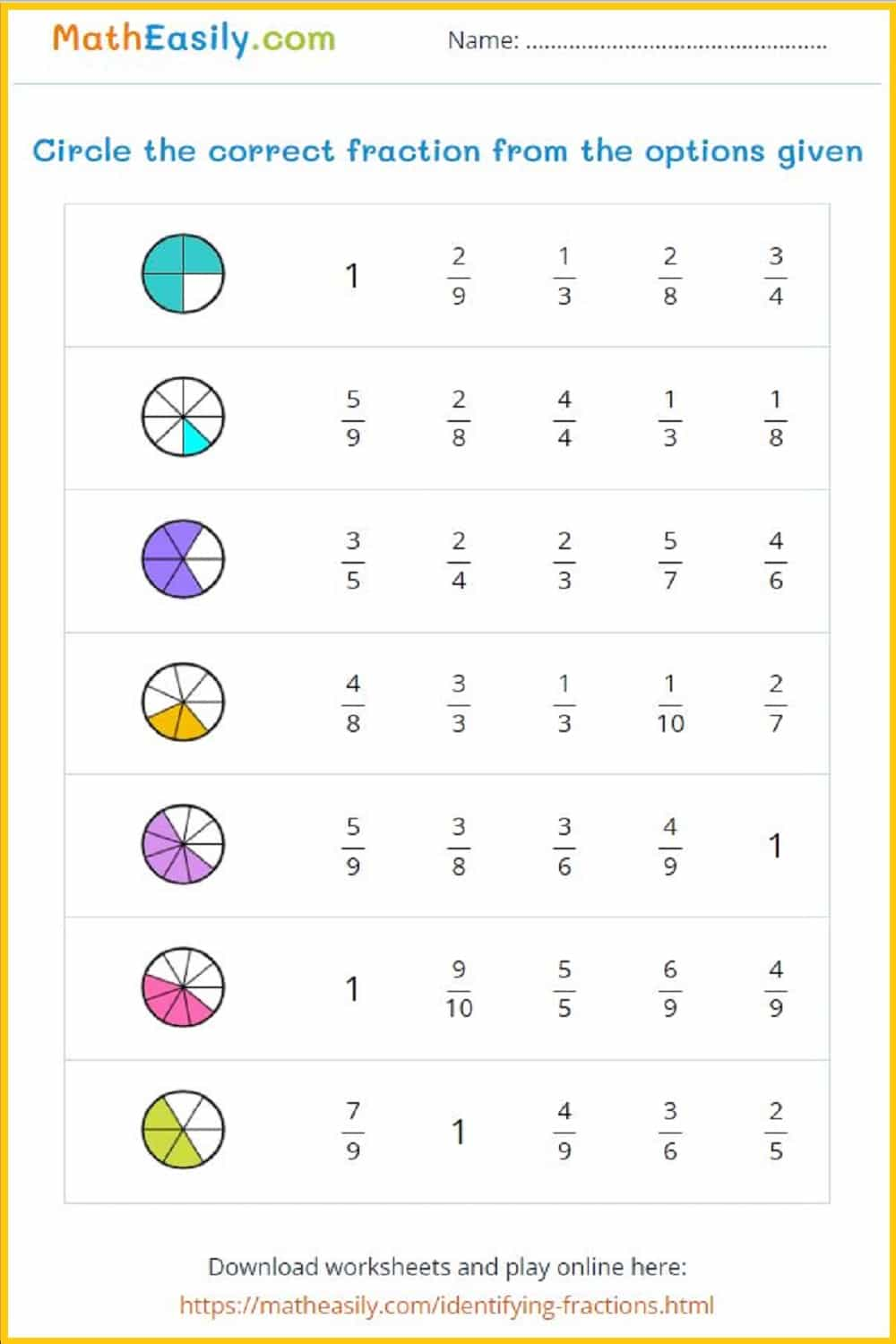Generate a new PDF

### Fraction flashcards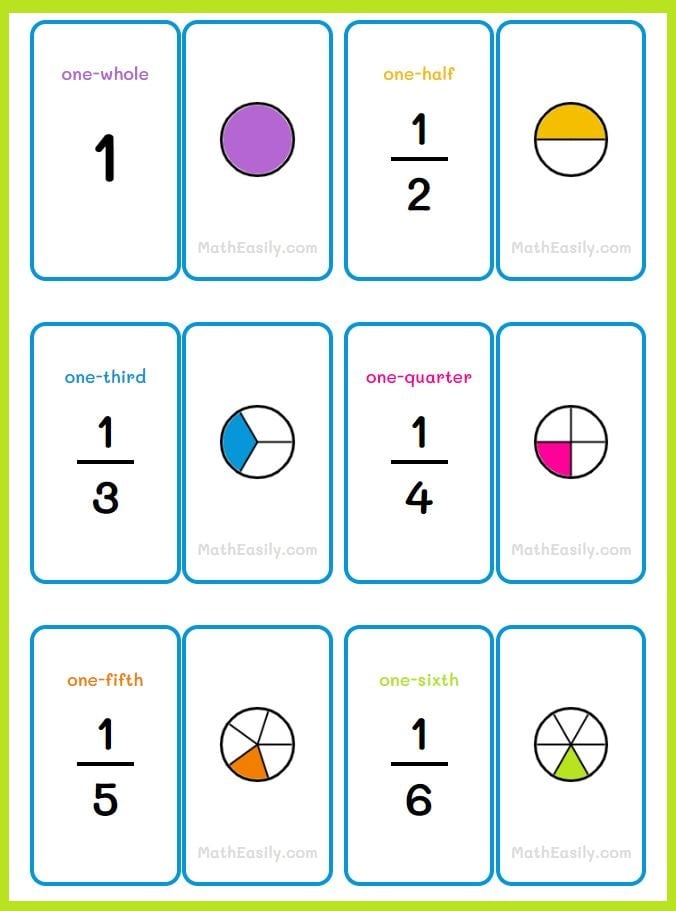### Fractions on number line

Identify fractions on a number line: worksheets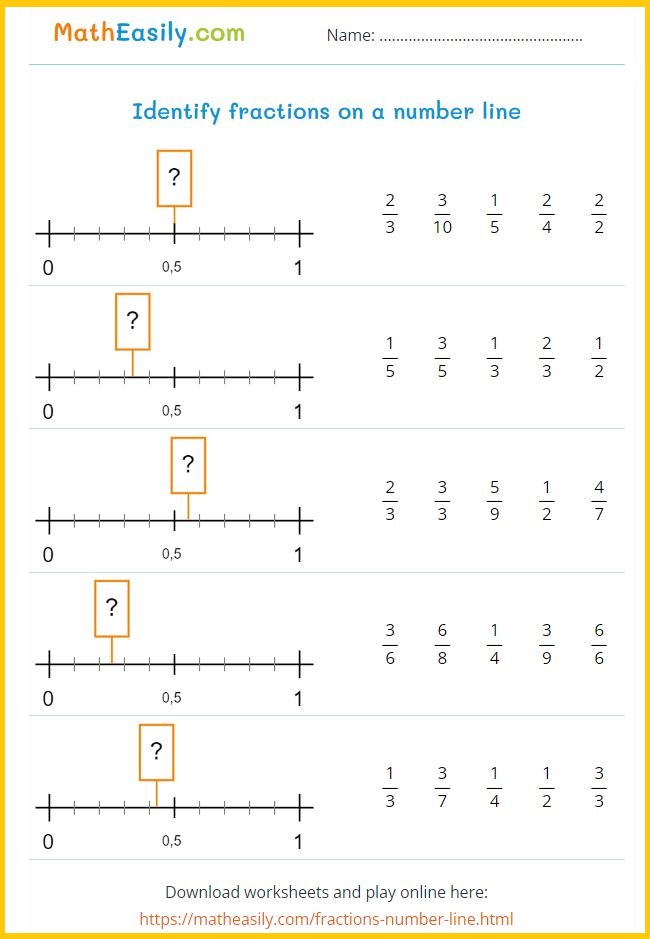Generate a new PDF

### Simplifying fractions

Simplifying fractions worksheets in PDF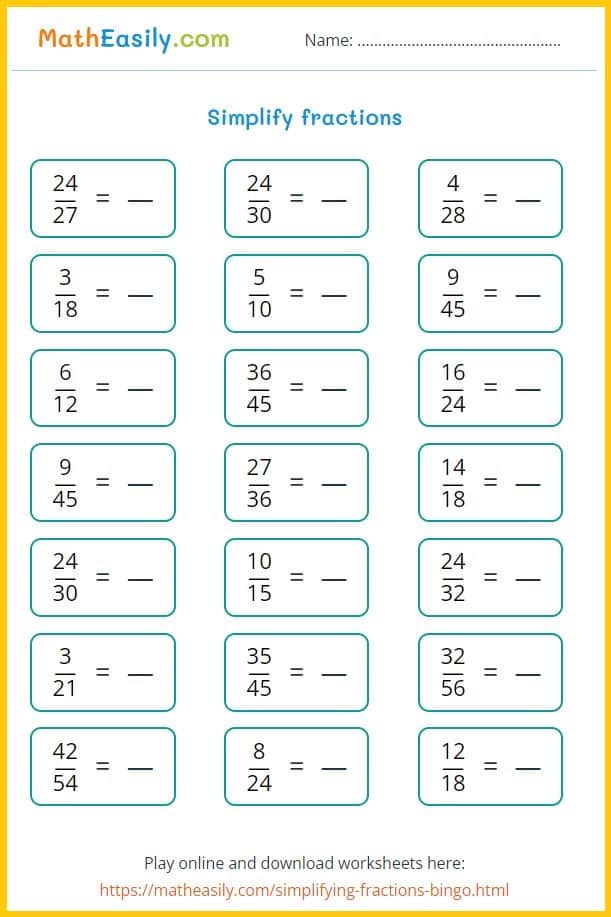Generate a new PDF

### Fractions BINGO

Printable simplifying fractions BINGO game with rules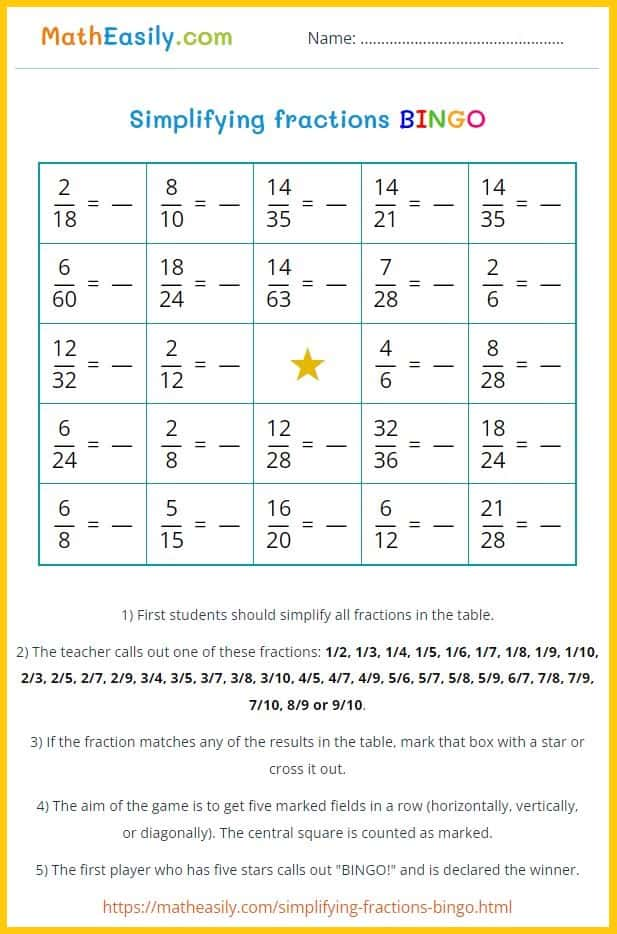Generate a new PDF

## Math Fraction Worksheets FREE

Our fractions worksheets allowing unlimited fraction practice opportunities. The advantages of automatically generated printable fractions worksheets include convenience, efficiency, and the ability to generate an unlimited number of unique fraction questions.

##### 100 Matchstick Puzzles in PDF

Printable math matchstick puzzles with answers. Move 1 stick to fix the equation.

US\$ 7.00

LOOK INSIDE

## Free Fraction Sheets PDF

Our printable fractions sheets can be used for both classwork and homework, and can help students develop their understanding of fractions. The difficulty level of the fraction worksheets can be adjusted according to the students' level of understanding and mastery of the topic.

These fraction worksheets can be used across several grade levels, typically from 3rd grade to 8th grade or higher, depending on the curriculum and educational system in use.

In the early grades, students are introduced to basic concepts such as recognizing fractions, understanding the meaning of numerator and denominator, and comparing and ordering fractions.

As students progress through the grades, they learn more advanced skills such as adding, subtracting, multiplying, and dividing fractions, working with mixed numbers, and solving word problems that involve fractions.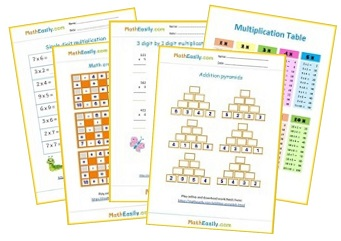Free Math Worksheets